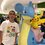# Sequences

I have an interesting sequence whose properties I do not know yet(that is why I'm asking for your help). Define $$a_{1} = 1$$ and $$a_{2} = 1$$ for this sequence. From then on, $$a_{n}$$ will be defined as $$a_{n-2} + a_{n-1}$$ if $$n \equiv 3 \mod {4}$$, $$a_{n-2} - a_{n-1}$$ if $$n \equiv 0 \mod {4}$$, $$a_{n-2} \times a_{n-1}$$ if $$n \equiv 1 \mod {4}$$, and $$a_{n-2} \div a_{n-1}$$ if $$n \equiv 2 \mod {4}$$. The first few terms of the sequence are $$1, 1, 2, -1, -2, \frac {1}{2}, -\frac {3}{2}, ...$$. I haven't found much, but I've hypothesized that similar sequences(mixing up the order of adding, subtracting, multiplying, and dividing) will result in a finite sequence(stopping when a term is undefined) if and only if a 0 appears(the iff is important). Anyone want to find something about this?Note by Tristan Shin
7 years, 2 months ago

This discussion board is a place to discuss our Daily Challenges and the math and science related to those challenges. Explanations are more than just a solution — they should explain the steps and thinking strategies that you used to obtain the solution. Comments should further the discussion of math and science.

When posting on Brilliant:

• Use the emojis to react to an explanation, whether you're congratulating a job well done , or just really confused .
• Ask specific questions about the challenge or the steps in somebody's explanation. Well-posed questions can add a lot to the discussion, but posting "I don't understand!" doesn't help anyone.
• Try to contribute something new to the discussion, whether it is an extension, generalization or other idea related to the challenge.
• Stay on topic — we're all here to learn more about math and science, not to hear about your favorite get-rich-quick scheme or current world events.

MarkdownAppears as
*italics* or _italics_ italics
**bold** or __bold__ bold
- bulleted- list
• bulleted
• list
1. numbered2. list
1. numbered
2. list
Note: you must add a full line of space before and after lists for them to show up correctly
paragraph 1paragraph 2

paragraph 1

paragraph 2

[example link](https://brilliant.org)example link
> This is a quote
This is a quote
    # I indented these lines
# 4 spaces, and now they show
# up as a code block.

print "hello world"
# I indented these lines
# 4 spaces, and now they show
# up as a code block.

print "hello world"
MathAppears as
Remember to wrap math in $$ ... $$ or $ ... $ to ensure proper formatting.
2 \times 3 $2 \times 3$
2^{34} $2^{34}$
a_{i-1} $a_{i-1}$
\frac{2}{3} $\frac{2}{3}$
\sqrt{2} $\sqrt{2}$
\sum_{i=1}^3 $\sum_{i=1}^3$
\sin \theta $\sin \theta$
\boxed{123} $\boxed{123}$

## Comments

Sort by:

Top Newest

Well, the sequence starting from $1,0,\ldots$ defies your conjecture.

It is a recursive sequence with repeated segment $[1,0,1,-1,-1]$

- 7 years, 1 month ago

Log in to reply

The sequence is defined with $a_2$ as $1$, but your sequence shows $a_2$ as $0$.

- 7 years, 1 month ago

Log in to reply

@Daniel Liu Your sequence has A2 as 0 but it is supposed to be 1

- 7 years, 1 month ago

Log in to reply

I don't see why $a_2$ can't be $0$. The OP said $a_1=a_2=1$, but I can surely change that, can I? Or can I only mix up the definitions for how to find later terms?

- 7 years, 1 month ago

Log in to reply

I meant only definitions for later terms. The first two terms must stay at 1, otherwise there are several contradictions(including yours).

- 7 years, 1 month ago

Log in to reply

×

Problem Loading...

Note Loading...

Set Loading...